Courses
Courses for Kids
Free study material
Offline Centres
More

# NCERT Exemplar for Class 11 Physics Chapter 11 - Thermal Properties of Matter (Book Solutions)Last updated date: 02nd Dec 2023
Total views: 612k
Views today: 17.12k

## NCERT Exemplar for Class 11 Physics - Thermal Properties of Matter - Free PDF Download

Free PDF download of NCERT Exemplar for Class 11 Physics  Chapter 11 - Thermal Properties of Matter solved by expert Physics teachers on Vedantu.com as per NCERT (CBSE) Book guidelines. All Chapter 11 - Thermal Properties of Matter Exercise questions with solutions to help you to revise complete syllabus and score more marks in your Examinations.

The NCERT Exemplar for the students of Class 11 has assembled all the important content that can explain the different Thermal properties of Matter in simple language. You can download the PDF version of the course materials from the website of Vedantu where you will get all the solutions to the questions. Go through the Chapters carefully in order to understand the laws explaining the various characteristics of Matters under different circumstances. As the final step of your preparation, solve the questions that are provided in the practice sets and recheck your answers.

## Access NCERT Exemplar Solutions for 11 Physics Chapter 11 -Thermal properties of matter

### MULTIPLE CHOICE QUESTIONS-I

11.1. A bimetallic strip is made of aluminium and steel ( ${{\alpha }_{Al}}>{{\alpha }_{steel}}$ ) . On heating, the strip will

(a) remain straight.

(b) get twisted.

(c) will bend with aluminium on the concave side.

(d) will bend with steel on the concave side.

Ans: The correct answer is option (d)

The bimetallic strip consists of steel and aluminium. When heated, the expansion of Aluminium is more than steel. Thus, steel will be on the concave side and aluminium on the convex side.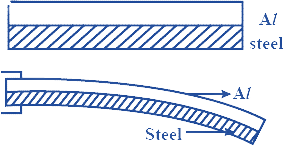11.2. A uniform metallic rod rotates about its perpendicular bisector with constant angular speed. If it is heated uniformly to raise its temperature slightly

(a) its speed of rotation increases.

(b) its speed of rotation decreases.

(c) its speed of rotation remains the same.

(d) its speed increases because its moment of inertia increases.

Ans: The correct answer is option (b)

Heating increases the length of the rod that will subsequently increase the moment of inertia from ${{I}_{1}}$ to ${{I}_{2}}$.

$\dfrac{{{I}_{1}}}{{{I}_{2}}}=\dfrac{{{\omega }_{2}}}{{{\omega }_{1}}}$

So, if ${{I}_{1}}$ is less than ${{I}_{2}}$ then ${{\omega }_{2}}$  will be less than ${{\omega }_{1}}$. Thus, (b) is correct.

11.3. The graph between two temperature scales A and B is shown in Figure. Between upper fixed point and lower fixed point there are 150 equal divisions on scale A and 100 on scale B. The relationship for conversion between the two scales is given by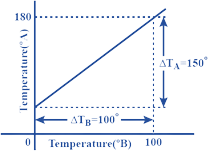(a) $\dfrac{{{t}_{A}}-180}{100}=\dfrac{{{t}_{B}}}{150}$

(b) $\dfrac{{{t}_{A}}-30}{150}=\dfrac{{{t}_{B}}}{100}$

(c) $\dfrac{{{t}_{A}}-30}{150}=\dfrac{{{t}_{A}}}{100}$

(d) $\dfrac{{{t}_{B}}-40}{100}=\dfrac{{{t}_{A}}}{180}$

Ans: The correct answer is option (b)

From the above graph:

Scale A:

Upper fixed point = ${{180}^{o}}$

Lower fixed point = ${{30}^{o}}$

Scale B:

Upper fixed point = ${{100}^{o}}$

Lower fixed point = ${{0}^{o}}$

Hence, the relation between scale A and B is given by,

$\dfrac{{{t}_{A}}-30}{150}=\dfrac{{{t}_{B}}-0}{100}$

$\dfrac{{{t}_{A}}-30}{150}=\dfrac{{{t}_{B}}}{100}$

11.4. An aluminium sphere is dipped into water. Which of the following is true?

(a) Buoyancy will be less in water at 0°C than that in water at 4°C.

(b) Buoyancy will be more in water at 0°C than that in water at 4°C.

(c) Buoyancy in water at 0°C will be the same as that in water at 4°C.

(d) Buoyancy may be more or less in water at 4°C depending on the radius of the sphere.

Ans: The correct answer is option (a)

The buoyant force at 0°C is given by,

$Fb=V{{\rho }_{0}}g$

And the buoyant force at 4°C is given by,

$F{{b}^{'}}=V{{\rho }_{4}}g$

$\dfrac{Fb}{F{{b}^{'}}}=\dfrac{{{\rho }_{0}}}{{{\rho }_{4}}}$

Density of water at 0°C is 0.998 g/cm3 and at 4°C is 1.000 gm/cm3.

$\dfrac{{{\rho }_{0}}}{{{\rho }_{4}}}<1$

$\dfrac{Fb}{F{{b}^{'}}}<1$

$Fb<F{{b}^{'}}$

Therefore, Buoyancy will be less in water at 0°C than that in water at 4°C.

11.5. As the temperature is increased, the time period of a pendulum,

(a) increases as its effective length increases even though its centre of mass still remains at the centre of the bob.

(b) decreases as its effective length increases even though its centre of mass still remains at the centre of the bob.

(c) increases as its effective length increases due to shifting of centre of mass below the centre of the bob.

(d) decreases as its effective length remains the same but the centre of mass shifts above the centre of the bob.

Ans: The correct answer is option (a)

The formula for time period of a pendulum is given by,

$T=2\pi \sqrt{\dfrac{L}{g}}$

$\therefore T\propto \sqrt{L}$

So, if the temperature increases, the pendulum's effective length (L) also increases even if the centre of mass is positioned at the centre of the bob.

11.6. Heat is associated with

(a) kinetic energy of random motion of molecules.

(b) kinetic energy of orderly motion of molecules.

(c) total kinetic energy of random and orderly motion of molecules.

(d) kinetic energy of random motion in some cases and kinetic energy of orderly motion in others.

Ans: The correct answer is option (a)

We know that when the temperature rises, the vibrations of molecules about their mean position rise as well. As a result, the kinetic energy associated with random molecular motion increases.

11.7. The radius of a metal sphere at room temperature T is R, and the coefficient of linear expansion of the metal is α. The sphere is heated a little by a temperature ΔT so that its new temperature is T+ ΔT. The increase in the volume of the sphere is approximately.

(a) $2\pi R\alpha \Delta T$

(b) $\pi R2\alpha \Delta T$

(c) $\dfrac{4\pi {{R}^{3}}\alpha \Delta T}{3}$

(d) $4\pi {{R}^{3}}\alpha \Delta t$

Ans: The correct answer is option (d)

Relation between Coefficient of cubical expansion ( $\gamma$ ) and linear expansion ( $\alpha$ ) is

$\gamma =3\alpha$

Volume = $\dfrac{4}{3}\pi {{R}^{3}}$

$\therefore \gamma =\dfrac{\Delta V}{V\Delta t}$

$\Delta V=\gamma V\Delta t$

$\Delta V=3\alpha \dfrac{4}{3}\pi {{R}^{3}}\Delta t$

$\Delta V=4\pi {{R}^{3}}\alpha \Delta t$

11.8. A sphere, a cube and a thin circular plate, all of the same material and same mass are initially heated to the same high temperature.

(a) Plate will cool fastest and cube the slowest

(b) Sphere will cool fastest and cube the slowest

(c) Plate will cool fastest and sphere the slowest

(d) Cube will cool fastest and plate the slowest.

Ans: The correct answer is option (c)

The following factors have a direct relationship with heat loss during cooling:

(i) temperature differential between the body and the environment

(ii) surface area exposed to the environment

(iii) Object's material

We know that the surface area of a sphere is the smallest, and the surface area of a circular plate is the largest. As a result, the sphere cools the slowest and the circular plate the fastest.

### MULTIPLE CHOICE QUESTIONS-II

11.9. Mark the correct options:

(a) A system X is in thermal equilibrium with Y but not with Z. System Y and Z may be in thermal equilibrium with each other.

(b) A system X is in thermal equilibrium with Y but not with Z. Systems Y and Z are not in thermal equilibrium with each other.

(c) A system X is neither in thermal equilibrium with Y nor with Z. The systems Y and Z must be in thermal equilibrium with each other.

(d) A system X is neither in thermal equilibrium with Y nor with Z. The system Y and Z may be in thermal equilibrium with each other.

Ans: The correct answer are options (b) and (d)

In this question, we'll use the Zeroth Law of Thermodynamics, which states that if two thermodynamic systems are both in thermal equilibrium with a third, they're both in thermal equilibrium.

Hence, if ${{T}_{x}}$ = ${{T}_{y}}$ , but ${{T}_{x}}$ $\ne $${{T}_{z}} , then {{T}_{y}} \ne {{T}_{z}} , then Y and Z are not in thermal equilibrium, so option (a) is incorrect. ${{T}_{x}}$ = {{T}_{y}} in option (b), but ${{T}_{x}}$ \ne {{T}_{z}} ,thus {{T}_{y}} \ne {{T}_{z}} , so this option is right. Option (c) is similarly incorrect since ${{T}_{x}}$ \ne {{T}_{y}} also ${{T}_{x}}$ \ne {{T}_{z}} , resulting in {{T}_{y}} \ne {{T}_{z}} Finally, in option (d), ${{T}_{x}}$ \ne$${{T}_{y}}$  and ${{T}_{x}}$ $\ne$ ${{T}_{z}}$ are equal, therefore ${{T}_{y}}$ may equal ${{T}_{z}}$ , making option (d) correct.

11.10. 'Gulab Jamuns' (assumed to be spherical) are to be heated in an oven. They are available in two sizes, one twice bigger (in radius) than the other. Pizzas (assumed to be discs) are also to be heated in the oven. They are also in two sizes, one twice bigger (in radius) than the other. All four are put together to be heated to oven temperature. Choose the correct option from the following:

(a) Both size gulab jamuns will get heated at the same time.

(b) Smaller gulab jamuns are heated before bigger ones.

(c) Smaller pizzas are heated before bigger ones.

(d) Bigger pizzas are heated before smaller ones.

Ans: The correct answer are options (b) and (c)

The smaller one has smaller thickness through which heat reaches the inside quickly as compared to the bigger ones. Hence option b and c are verified.

11.11. Refer to the plot of temperature versus time (Figure) showing the changes in the state of ice on heating (not to scale). Which of the following is correct?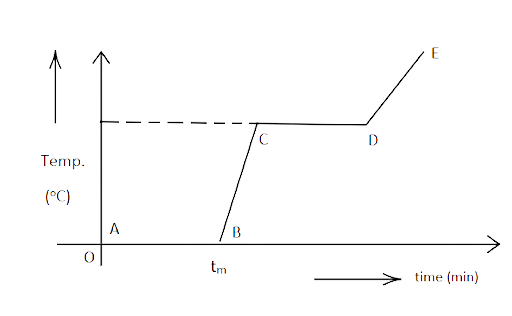(a) The region AB represents ice and water in thermal equilibrium.

(b) At B water starts boiling.

(c) At C all the water gets converted into steam.

(d) C to D represents water and steam in equilibrium at boiling point.

Ans: The correct answer are options (a) and (d)

When a material heats up but does not change its temperature, its condition (solid, liquid, or gas) changes. As a result, parts AB and CD (1000C) reflect solid to liquid and liquid to gas conversions, respectively. Part AB denotes ice and water up to B, whereas part CD denotes water and steam.

11.12. A glass full of hot milk is poured on the table. It begins to cool gradually. Which of the following is correct?

(a) The rate of cooling is constant till milk attains the temperature of the surrounding.

(b) The temperature of milk falls off exponentially with time.

(c) While cooling, there is a flow of heat from milk to the surrounding as well as from surrounding to the milk but the net flow of heat is from milk to the surrounding and that is why it cools.

(d) All three phenomena, conduction, convection and radiation are responsible for the loss of heat from milk to the surroundings.

Ans: The correct answer are options (b), (c) and (d)

When hot milk is put on a table, it radiates heat across the room. Option d is verified by conduction, convection, and radiation. The heat of milk decreases exponentially as a result of Newton's law of cooling (verifies option (b)).

Heat loss is related to the temperature differential between the environment and the body. As the temperature differential between the milk and the air diminishes, the rate of cooling reduces with time (reject option (a)).

When the milk cools, a tiny quantity of heat flows from the surrounding to the milk, compared to the heat lost by the milk to the surrounding (confirms option (c)).

### VERY SHORT ANSWER TYPE QUESTIONS

11.13. Is the bulb of a thermometer made of diathermic walls or adiabatic walls?

Ans: Adiabatic walls prevent heat from passing into the bulb's mercury, but diathermic walls permit heat to pass through. As a result, diathermic walls are employed in thermometer bulbs.

11.14. A student records the initial length l, change in temperature ΔT and change in length Δl of a rod as follows:

 Sr. No. l(m) ΔT (oC) Δl (m) 1 2 10 $4\times {{10}^{-4}}$ 2 1 10 $4\times {{10}^{-4}}$ 3 2 10 $2\times {{10}^{-4}}$ 4 3 10 $6\times {{10}^{-4}}$

If the first observation is correct, what can you say about observations 2, 3 and 4.

Ans: As per the 1st observation we can infer that linear expansion $\alpha$ is,

$\alpha =\dfrac{\Delta l}{l\Delta t}=\dfrac{4\times {{10}^{-4}}}{2\times 10}=2\times {{10}^{-5}}^{o}{{C}^{-1}}$

For observation no. 2,

$\alpha =\dfrac{\Delta l}{l\Delta t}$

$\Delta l=\alpha l\Delta t=2\times {{10}^{-5}}\times 1\times 10=2\times {{10}^{-4}}m$

But given value is Δl = $4\times {{10}^{-4}}m$

So, 2nd observation is incorrect.

For observation no. 3,

$\alpha =\dfrac{\Delta l}{l\Delta t}$

$\Delta l=\alpha l\Delta t=2\times {{10}^{-5}}\times 2\times 20=8\times {{10}^{-4}}m$

But given value is Δl = $2\times {{10}^{-4}}m$

So, 3rd observation is incorrect.

For observation no. 4,

$\alpha =\dfrac{\Delta l}{l\Delta t}$

$\Delta l=\alpha l\Delta t=2\times {{10}^{-5}}\times 3\times 10=6\times {{10}^{-4}}m$

Here the obtained value is the same as the given value.

So, the 4th observation is correct.

11.15. Why does a metal bar appear hotter than a wooden bar at the same temperature? Equivalently it also appears cooler than wooden bars if they are both colder than room temperature.

Ans: (i) It is owing to the fact that metal bars have a far higher conductivity than wood. As a result, the rate of heat transmission in metal is much faster than in wood.

(ii) Because metal has a low specific heat as compared to wood, it takes far less heat to change a degree of temperature in metal than it does in wood.

As a result of their higher conductivity and lower specific heat, metals grow colder in colder environments and hotter in hotter environments than wood.

11.16. Calculate the temperature which has the same numeral value on Celsius and Fahrenheit scale.

Ans: Let the required temperature be ${{x}^{o}}C$ and ${{x}^{o}}F$

The numeral value has to be equal.

$\therefore \dfrac{C}{100}=\dfrac{F-32}{180}$

$\therefore \dfrac{x}{100}=\dfrac{x-32}{180}$

Multiplying both the sides by 20 we get,

$\therefore \dfrac{x}{5}=\dfrac{x-32}{9}$

$x\times 9=5\times \left( x-32 \right)$

$9x=5x-160$

$4x=-160$

$x=-40$

$\therefore -{{40}^{o}}C=-{{40}^{o}}F$

11.17. These days' people use steel utensils with copper bottoms. This is supposed to be good for uniform heating of food. Explain this effect using the fact that copper is the better conductor.

Ans: Copper has a higher conductivity and a lower specific heat than steel. Because copper has a lower specific heat than steel, it transfers heat fast from the bottom to the food and requires less heat. As a result, the copper base transfers heat from the burner to the food in the utensil quickly and efficiently.

11.18. Find out the increase in moment of inertia I of a uniform rod (coefficient of linear expansion α) about its perpendicular bisector when its temperature is slightly increased by ΔT.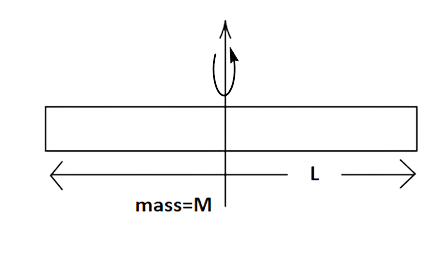Ans: The relation between moment of inertia, mass and length is,

Moment of inertia along the perpendicular bisector = $\dfrac{1}{12}M{{L}^{2}}$

$\Delta L=\alpha L\Delta T$

$I\prime =\dfrac{1}{2}M{{(L+\Delta L)}^{2}}=\dfrac{1}{2}M({{L}^{2}}+\Delta {{L}^{2}}+2L\Delta L)$

$\Delta {{L}^{2}}$ is a negligibly small term

$\Rightarrow I\prime =\dfrac{M}{12}({{L}^{2}}+2L\Delta L)$

$\Rightarrow \text{ }=\dfrac{M{{L}^{2}}}{12}+\dfrac{ML\Delta L}{6}\times \dfrac{2L}{2L}$

$\Rightarrow \text{ }=\dfrac{M{{L}^{2}}}{12}+\dfrac{M{{L}^{2}}}{12}\times \dfrac{2\Delta L}{2L}$

$\Rightarrow I+I\times \dfrac{2\alpha L\Delta T}{L}$

$\therefore {{I}^{'}}=I+2\alpha \Delta T$

11.19. During summers in India, one of the common practices to keep cool is to make ice balls of crushed ice, dip it in flavoured sugar syrup and sip it. For this a stick is inserted into crushed ice and is squeezed in the palm to make it into the ball. Equivalently in winter, in those areas where it snows, people make snow balls and throw them around. Explain the formation of balls out of crushed ice or snow in the light of P–T diagram of water.

Ans: Water is represented as a P – T graph or diagram with a double headed arrow. When the temperature is 0oC and the pressure is 1 atm, ice becomes liquid, and when the temperature is 0oC and the pressure is 1 atm, water becomes ice.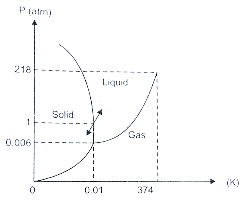When crushed ice is pressed, some of it melts into water at 0 degrees Celsius, filling the crevices between the ice flakes. The M.P. grew as the ice flakes were squeezed, and water between the flakes also froze into ice, binding all of the ice flakes together and making the ball more stable.

11.20. 100 g of water is supercooled to –10°C. At this point, due to some disturbance mechanized or otherwise some of it suddenly freezes to ice. What will be the temperature of the resultant mixture and how much mass would freeze?

$[{{S}_{w}}=1cal/g{{/}^{o}}C\text{ }and\text{ }{{\text{L}}^{w}}_{fusion}=80cal/g]$

Ans: Given mass of water = 100gm.

The mixture of ice and water exists at –10°C.

Heat emitted = $ms\Delta t=100\times 1\times [0-(-10)]cal=1000cal$

$Q=ms\Delta t=100\times 1\times [0-(-10)]cal=1000cal$

Mass of melted ice = $\dfrac{Q}{l}=\dfrac{1000}{80}=12.5gm$

Mass of water that froze is 12.5 grams.

11.21. One day in the morning, Ramesh filled up 1/3 a bucket of hot water from a geyser, to take a bath. Remaining 2/3 was to be filled by cold water (at room temperature) to bring the mixture to a comfortable temperature. Suddenly Ramesh had to attend to something which would take some time, say 5-10 minutes before he could take a bath. Now he had two options: (i) fill the remaining bucket completely by cold water and then attend to the work, (ii) first attend to the work and fill the remaining bucket just before taking a bath. Which option do you think would have kept water warmer? Explain.

Ans: According to Newton's law of cooling, the rate of cooling or heat energy loss is proportional to the temperature differential between the body and its surroundings. As a result, the first alternative would be to keep the water warmer, because the temperature differential between the surrounding and the water is tiny. As a result, the first choice has a lower quantity of heat energy loss. Because the differential between the water and the surrounding is high in the second choice, a greater amount of heat energy is released.

11.22. We would like to prepare a scale whose length does not change with temperature. It is proposed to prepare a unit scale of this type whose length remains, say 10 cm. We can use a bimetallic strip made of brass and iron each of different length whose length (both components) would change in such a way that the difference between their lengths remains constant. If ${{\alpha }_{iron}}=1.2\times {{10}^{-5}}/K$ and ${{\alpha }_{brass}}=1.8\times {{10}^{-5}}/K\text{ }$ what should we take as length of each strip?

Ans: At any temperature, the required scale may be created using iron and brass rods with one end aligned with A and the other ends separated where PB=10 cm.

Let the initial length of brass be ${{L}_{1brass}}$ and of iron be ${{L}_{1iron}}$

${{L}_{1iron}}-{{L}_{1brass}}=10...(i)$

We know that,

$\therefore \alpha =\dfrac{\Delta L}{{{L}_{0}}\Delta T}=\dfrac{{{L}_{2}}-{{L}_{1}}}{{{L}_{1}}\Delta T}$

${{L}_{2}}={{L}_{1}}={{L}_{1}}\alpha \Delta T$

${{L}_{2}}={{L}_{1}}(1+\alpha \Delta T)$

The combination of the rods are again heated then the length changes to ${{L}_{2brass}}$ for brass ${{L}_{2iron}}$ for iron.

As per the question,

${{L}_{2iron}}-{{L}_{2brass}}=10$

$\Rightarrow {{L}_{1iron}}(1+{{\alpha }_{1}}\Delta T)-{{L}_{1brass}}(1+{{\alpha }_{2}}\Delta T)=10$

$\Rightarrow {{L}_{1iron}}-{{L}_{1brass}}({{\alpha }_{1}}{{L}_{1iron}}-{{\alpha }_{2}}{{L}_{1brass}})\Delta T=10$

$\Rightarrow 10({{\alpha }_{1}}{{L}_{1iron}}-{{\alpha }_{2}}{{L}_{1brass}})\Delta T=10....[from(i)]$

$\Rightarrow {{\alpha }_{1}}{{L}_{1iron}}={{\alpha }_{2}}{{L}_{1brass}}$

$\Rightarrow \dfrac{{{L}_{1iron}}}{{{L}_{1brass}}}=\dfrac{{{\alpha }_{2}}}{{{\alpha }_{1}}}=\dfrac{1.8\times {{10}^{-5}}/K\text{ }}{1.2\times {{10}^{-5}}/K\text{ }}=\dfrac{18}{12}=\dfrac{3}{2}$

$\Rightarrow \dfrac{{{L}_{1iron}}}{{{L}_{1brass}}}=\dfrac{3}{2}$

Let x be the common multiple.

Thus, ${{L}_{1iron}}$ = 3x and ${{L}_{1brass}}$ = 2x.

$3x-2x=10$

x = 10

${{L}_{1iron}}$ = 3x = $3\times 10=30cm$

and ${{L}_{1brass}}$ = 2x = $2\times 10=20cm$

the length of each strip should be 30 cm and 20 cm.

11.23. We would like to make a vessel whose volume does not change with temperature (take a hint from the problem above). We can use brass and iron ${{\beta }_{\upsilon brass}}\text{ }=\text{ }6\times {{10}^{5}}/K$ and ${{\beta }_{\upsilon iron}}\text{ }=\text{ 3}\text{.55}\times {{10}^{5}}/K$ to create a volume of 100 cc. How do you think you can achieve this?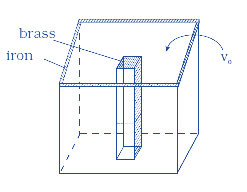Ans: We're building a jar in which the volume of Brass does not vary with temperature.

We'll need an iron vessel with a brass rod inside to build the necessary vessel, as illustrated in the diagram.

Let volume of vessel be ${{V}_{0}}$ , iron vessel be ${{V}_{iron}}$ and brass rod be ${{V}_{B}}$

${{V}_{0}}=100cc={{10}^{-4}}{{m}^{3}}$

${{V}_{0}}=~{{V}_{iron}}-{{V}_{B}}={{10}^{-4}}{{m}^{3}}$

Now,

${{\beta }_{iron}}{{V}_{iron}}\Delta T={{\beta }_{B}}{{V}_{B}}\Delta T$

$\Rightarrow {{V}_{iron}}=\dfrac{{{\beta }_{B}}{{V}_{B}}}{{{\beta }_{iron}}}=\left( \dfrac{6\times {{10}^{5}}/K}{3.55\times {{10}^{5}}/K} \right){{V}_{B}}$

$\Rightarrow {{V}_{iron}}=1.69{{V}_{B}}$

We know that,

${{V}_{iron}}-{{V}_{B}}={{10}^{-4}}{{m}^{3}}$

$\Rightarrow 1.69{{V}_{B}}-{{V}_{B}}={{10}^{-4}}{{m}^{3}}$

$\Rightarrow 0.69{{V}_{B}}={{10}^{-4}}{{m}^{3}}$

$\Rightarrow {{V}_{B}}=\dfrac{{{10}^{-4}}{{m}^{3}}}{0.69}=144.9c{{m}^{3}}$

Also,

$\Rightarrow {{V}_{iron}}=1.6\times 144.9=244.9c{{m}^{3}}$

As a result, an iron vessel with a volume of 249.9 $c{{m}^{3}}$ and a brass rod with a volume of 144.9 $c{{m}^{3}}$ will function as a vessel with a volume of 100 $c{{m}^{3}}$ that will not vary with temperature.

11.24. Calculate the stress developed inside a tooth cavity filled with copper when hot tea at temperature of $\mathbf{5}{{\mathbf{7}}^{\mathbf{o}}}\mathbf{C}$ is drunk. You can take body (tooth) temperature to be $\mathbf{3}{{\mathbf{7}}^{\mathbf{o}}}\mathbf{C}$ and $\mathbf{\alpha }=\mathbf{1}.\mathbf{7}\times \text{ }\mathbf{1}{{\mathbf{0}}^{-\mathbf{5}}}/\mathbf{K}$ . Bulk modulus for copper = $\mathbf{140}\text{ }\times \mathbf{1}{{\mathbf{0}}^{\mathbf{9}}}\mathbf{N}/{{\mathbf{m}}^{\mathbf{2}}}$ .

Ans: Given:

Temperature difference = ∆t = 57 – 37 =  ${{20}^{o}}C$

Bulk modulus for copper (B) = $140 \times {{10}^{9}} \,N/{m^2}$

$\alpha \text{ }=\text{ }1.7\text{ }\times \text{ }{{10}^{-5}}/{}^\circ C$

$\gamma \text{ }=\text{ }3\alpha \text{ }=\text{ }5.1\text{ }\times \text{ }{{10}^{-5}}^{o}C$

$Thermal\text{ }stress=B\left( \gamma \Delta t \right)=\text{140}\times {{10}^{9}}(5.1\times {{10}^{-5}}^{o}C\times {{20}^{o}}C)=1.428\text{ }\times \text{ }108\text{ }N/{{m}^{2}}$

11.25. A rail track made of steel having length 10 m is clamped on a railway line at its two ends (Figure). On a summer day due to a rise in temperature by ${{20}^{o}}C$ , it is deformed as shown in figure. Find x (displacement of the centre) if ${{\mathbf{\alpha }}_{\mathbf{steel}}}=\mathbf{1}.\mathbf{2}\times \mathbf{1}{{\mathbf{0}}^{-\mathbf{5}}}/{}^\circ \mathbf{C}$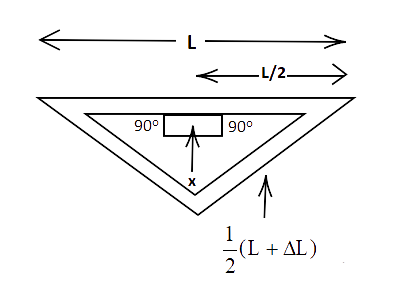Ans: Given:

$\Delta t={{20}^{o}}C$

${{\alpha }_{steel}}\text{ }=\text{ }1.2\times \text{ }{{10}^{-5}}/{}^\circ C.$

${{L}_{c}}=10m$

For displacement (x) we will use Pythagoras theorem,

${{x}^{2}}={{\left( \dfrac{1}{2}\left( L+\Delta L \right) \right)}^{2}}-{{\left( \dfrac{L}{2} \right)}^{2}}$

$=\dfrac{1}{4}[{{L}^{2}}+\Delta {{L}^{2}}+2L\Delta L]-4{{L}^{2}}$

$=\dfrac{{{L}^{2}}}{4}+{{\dfrac{\Delta L}{4}}^{2}}+\dfrac{2L\Delta L}{4}-\dfrac{{{L}^{2}}}{4}$

$\Delta {{L}^{2}}$ is a very small quantity so it can be neglected

${{x}^{2}}=\dfrac{2L\Delta L}{4}$

${{x}^{2}}=\dfrac{1}{2}\sqrt{2L\Delta L}$

$\Delta L={{L}_{c}}\times \alpha \times \Delta t=240.0\times {{10}^{-5}}$

Substituting this value in the above formula we get,

$x=\dfrac{1}{2}\sqrt{2\times 10\times 240.0\times {{10}^{-5}}}=10.8m$

Therefore, the displacement x is 10.8m.

11.26. A thin rod having length ${L_0}$ at $\mathbf{0}{}^\circ \mathbf{C}$ and coefficient of linear expansion α has its two ends maintained at temperatures ${{\theta }_{1}}\text{ and }{{\theta }_{2}}$ , respectively. Find its new length.

Ans: The temperature of the rod increases from ${{\theta }_{1}}\text{ to }{{\theta }_{2}}$ one end to another. Let the mean temperature of the rod be $\theta$

Thus, $\theta$ = $\dfrac{{{\theta }_{1}}+{{\theta }_{2}}}{2}$

We know that,

$L={{L}_{0}}\left( 1+\alpha \theta \right)={{L}_{0}}\left( 1+\alpha \left( \dfrac{{{\theta }_{1}}+{{\theta }_{2}}}{2} \right) \right)$

11.27. According to Stefan's law of radiation, a black body radiates energy from its unit surface area every second where T is the surface temperature of the black body and $\sigma =5.67\times {{10}^{8}}W/{{m}^{2}}/{{K}^{4}}$ is known as Stefan's constant. A nuclear weapon may be thought of as a ball of radius 0.5 m. When detonated, it reaches a temperature of ${{10}^{6}}K$  and can be treated as a black body.

(a) Estimate the power it radiates.

Ans. As per Stefan's law of radiation, a black body radiates the energy from its unit surface area every second.

(a) Given: R = 0.5m, $\sigma =5.67\times {{10}^{8}}W/{{m}^{2}}/{{K}^{4}}$ , T = ${{10}^{6}}K$

Here,

$E=\sigma {{T}^{4~}}per\text{ second/per sq}\text{. m}$

Power radiated per sec (P) = AE = A $\sigma {{T}^{4~}}$

$P=\left( 4\pi {{R}^{2}} \right)\times 5.67\times {{10}^{8}}W/{{m}^{2}}/{{K}^{4}}\times {{({{10}^{6}})}^{4}}K$

$P=\left( 4\times 3.14\times {{\left( 0.5 \right)}^{2}} \right)\times 5.67\times {{10}^{16}}$

$P=18\times {{10}^{16}}=1.8\times {{10}^{17}}$

(b) If the surrounding has water at ${{30}^{o}}C$, how much water can 10% of the energy produced evaporate in 1 s?

$\left[ {{S}_{w}}=4186.0J/kgK,\text{ }{{L}_{v}}=22.6\times {{10}^{5}}J/kg \right]$

Ans. $P=1.8\times {{10}^{17}}$

To evaporate water 10% of this power is utilised.

$E=\dfrac{10}{100}\times 1.8\times {{10}^{17}}=1.8\times {{10}^{16}}J/s$

The energy needed by water to evaporate from ${{30}^{o}}C\text{ to 10}{{0}^{o}}C$ = E used to heat up from ${{30}^{o}}C\text{ to 10}{{0}^{o}}C$ + E used to evaporate water into vapour.

$\Rightarrow m{{S}_{w}}({{T}_{2}}-{{T}_{1}})+mL=m({{S}_{w}}({{T}_{2}}-{{T}_{1}})+L)$

$\Rightarrow 1.8\times {{10}^{16}}=m[4180(100-30)+22.6\times {{10}^{5}}$

$\Rightarrow m(2.93020+22.6)\times {{10}^{5}}=1.8\times {{10}^{16}}$

$\Rightarrow m(25.6)\times {{10}^{5}}=1.8\times {{10}^{16}}$

$\Rightarrow m=\dfrac{1.8\times {{10}^{16}}}{(25.6)\times {{10}^{5}}}=7\times {{10}^{9}}kg$

(c) If all this energy U is in the form of radiation, corresponding momentum is $p=\dfrac{U}{c}$ . How much momentum per unit time does it impact on the unit area at a distance of 1 km?

Ans. Given:

$p=\dfrac{U}{c}=\dfrac{1.8\times {{10}^{17}}}{3\times {{10}^{8}}}=6\times {{10}^{9}}$

But, $P=1.8\times {{10}^{17}}$

Thus, power per unit time/ unit area = $\dfrac{6\times {{10}^{9}}}{4\pi {{R}^{2}}}=\dfrac{6\times {{10}^{9}}}{4\times 3.14\times {{\left( {{10}^{3}} \right)}^{2}}}=\dfrac{6\times 100}{12.56}=47.77Kgm/{{s}^{2}}/{{m}^{2}}$

$P/\sec \text{ at 1 km on }{{m}^{2}}\cong 47.8Kgm/{{s}^{2}}/{{m}^{2}}$

Various revision notes are also there on the website to help you in revising. Solving the questions will not only help you to get an idea of how many questions you can correctly attempt but you will get to learn how much you are prepared to appear for the Examinations.

If you are not sure how much you need to cover to score good marks in your Examination, then you can have a look at the content of the NCERT Exemplar as all the important Chapters are there that you need to study.

The laws of Physics that explain the different kinds of Thermal properties of Matter in detail are elaborated in the NCERT Exemplars with Examples that will help the students to connect with the concept. Register for the free Classes to know more and you can also register for the one-on-one Classes that are available in the premium package.

## FAQs on NCERT Exemplar for Class 11 Physics Chapter 11 - Thermal Properties of Matter (Book Solutions)

1. What does the Thermal property of Matters signify?

A Physical Matter can have a number of properties that determine its state and behaviorism. The Thermal property of a particular Matter signifies the state and characteristics of the particular Matter under a particular temperature range. When heat is conducted through a particular Matter, the behaviorism and the properties of that particular Matter that we can perceive are known as Thermal properties. There are various components of Thermal properties that can be further explained by the laws of Physics.

2. What is the relationship between the Thermal properties and the temperature of a particular Matter?

A Physical Matter can be present in various types of states. The form of state in which a particular Matter exists is further determined by the positions of various particles that it is constituted of and by the physical properties of the Matter. The Matter can show distinguishable characteristics if we can alter the temperature ranges. There are various physical laws that can explain the change of behaviorism of Matter when it is placed under different temperature ranges or when heat passes through the particles of Matter.

3. How many types of Thermal properties are there?

The various types of Thermal properties explain the reasons for the different kinds of behaviorism and characteristics displayed by a particular Matter when heat passes through them. For Example, heat capacity defines one of the physical properties of Matter. It refers to the amount of heat that is needed to pass through the particles of a Matter in order to produce a specific amount of change in the temperature. Another physical property of a Matter is known as Thermal Expansion and it denotes the change of the physical and outward properties of the Matter like the shape, volume, density, etc. Thermal conductivity explains the amount of energy produced in a particular Matter due to the movement of the molecules and particles under a particular temperature range.

4. How many types of Thermal Expansion are there?

The term itself explains the significance of this particular physical property of Matters. If the Matter is placed under a definite range of temperature or a specific amount of heat is transmitted through the molecules and particles of the Matter, their outward properties will change and hence the characteristics of the Matter will also be altered. Three types of Thermal Expansion are known. Depending on the particular dimension of the Matters where the changes take place, there can be three types of Thermal Expansions and they are areal Expansion, linear and volumetric Expansion.

5. What is the significance of understanding the concept of Thermal properties of Matter?

The concept is introduced by CBSE to the students of Class 11 and the Thermal properties of Matter are considered one of those foundational concepts of Physics. It helps the students to understand and acquire a detailed knowledge of the various properties of Matters including the Thermal properties of a particular Matter. By understanding these concepts the students can explain the reasons why a particular Matter changes its characteristics when it is placed under different ranges of temperature or heat passes through it. The laws of Physics also explain what can be the characteristics displayed by the Matter under different temperature ranges. To know more you can visit the Vedantu website to get all the important course materials for even better preparation.